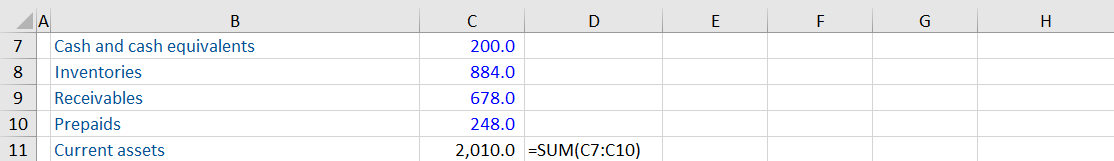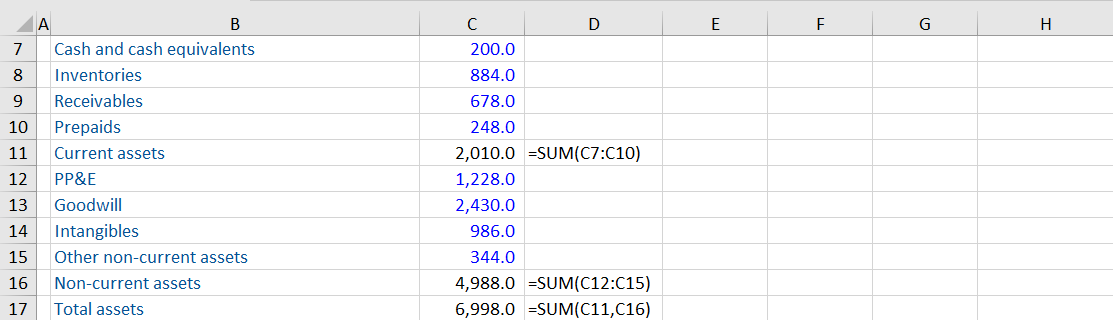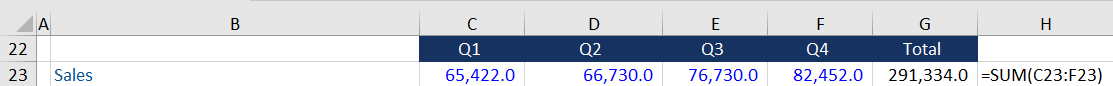## What is the SUM function?

The SUM function is a very sophisticated function that allows you to add multiple values at once. It is worth knowing exactly how it is coded as data can be set up in order to maximize its usefulness. The ALT = keyboard shortcut activates the SUM function.

The function coding assumes you are working from the top left to bottom right so is built to work vertically and horizontally. The numbers above its location are included in the addition (vertically). You can insert a blank row or a text header and be confident that ALT = will add the data immediately above until it reaches the inserted text header or black row.

### Syntax

The syntax for this function is shown as:

SUM(number1,[number 2,…number_n])

Or

SUM(cell1:cell2,[cell3:cell4],…)

## Example

In the following example, the SUM function shortcut was activated with the cursor in cell C11 and it is assumed that the required addition was C7 through to C10. It did so because C6 is a blank cell. Activating the keyboard shortcut, in this instance, meant that Excel automatically inserted SUM=(C7:C10).You should always check at this stage that the selection is appropriate and, once comfortable, process the sum by clicking Enter on your keyboard.

In the illustration below, the cursor is in cell C16 when the SUM function shortcut is activated. It adds all of the data in the range of cells above but, in this case, it stops at cell C12. However, cell C11 does have a value, so why has it been excluded? The presence of a SUM function in C11 means that Excel recognizes it as a subtotal and assumes (correctly in this case) that it is to be excluded. This highlights the importance of checking prior to pressing Enter to process because the selection chosen by Excel might not be what is intended by the model builder.The following illustration indicates that the SUM function shortcut has been activated with the cursor in cell C17. Excel has guessed correctly that the sum should use the data that is above cell C17. However, it has chosen the prior two subtotals for current assets and non-current assets. This is because the formula immediately above is a SUM function. As a consequence, Excel assumes the user wants to sum the subtotals. In this case, current and non-current assets are summed to arrive at total assets.Finally, Excel’s default assumption is that the data to be added is above the answer cell and it will always check this first. If there is a text header or a blank row immediately above the answer cell, then it will assume that the user wants to add from the left; therefore, it will check values to the left of the answer cell. In the illustration below, the answer cell is in G23 and there is a text header in B23, which means when the SUM shortcut is activated, Excel includes the data from C23 to F23.